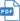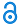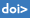# Cornelia Schiebold

Professor

• Professional title: Professor
• Department: Department of Engineering, Mathematics and Science Education (IMD)
• Telephone: +46 (0)10-1428462
• Email: cornelia.schiebold@miun.se
• Location: Sundsvall
• Employee in the subject: Mathematics

## Publications

### Articles in journals

Nilson, T. & Schiebold, C. (2020). Solution formulas for the two-dimensional Toda lattice and particle-like solutions with unexpected asymptotic behaviour. Journal of Nonlinear Mathematical Physics, vol. 27: 1, pp. 57-94.Carillo, S. , Schiavo, M. L. & Schiebold, C. (2019). Abelian versus non-Abelian Bäcklund charts : Some remarks. Evolution Equations and Control Theory, vol. 8: 1, pp. 43-55.Carillo, S. , Lo Schiavo, M. , Porten, E. & Schiebold, C. (2018). A novel noncommutative KdV-type equation, its recursion operator, and solitons. Journal of Mathematical Physics, vol. 59: 4Carillo, S. , Lo Schiavo, M. & Schiebold, C. (2018). Recursion operators admitted by non-Abelian Burgers equations : some remarks. Mathematics and Computers in Simulation, vol. 147: SI, pp. 40-51.Schiebold, C. (2017). Asymptotics for the multiple pole solutions of the nonlinear Schrödinger equation. Nonlinearity, vol. 30: 7, pp. 2930-2981.Li, S. , Biondini, G. & Schiebold, C. (2017). On the degenerate soliton solutions of the focusing nonlinear Schrödinger equation. Journal of Mathematical Physics, vol. 58: 3Carillo, S. , Lo Schiavo, M. & Schiebold, C. (2016). Bäcklund transformations and non-abelian nonlinear evolution equations : A novel bäcklund chart. SIGMA. Symmetry, Integrability and Geometry, vol. 12Carillo, S. & Schiebold, C. (2012). On the recursion operator for the noncommutative Burgers hierarchy. Journal of Nonlinear Mathematical Physics, vol. 19: 1, pp. Art. no. 1250003Carillo, S. & Schiebold, C. (2011). Matrix Korteweg-de Vries and modified Korteweg-de Vries hierarchies : Noncommutative soliton solutions. Journal of Mathematical Physics, vol. 52: 5, pp. Art. no. 053507Schiebold, C. (2011). Structural properties of the noncommutative KdV recursion operator. Journal of Mathematical Physics, vol. 52: 11, pp. Art. no. 113504Schiebold, C. (2010). Cauchy-type determinants and integrable systems. Linear Algebra and its Applications, vol. 433: 2, pp. 447-475.Schiebold, C. (2010). The noncommutative AKNS system: projection to matrix systems, countable superposition and soliton-like solutions. Journal of Physics A, vol. 43: 43, pp. 434030Carillo, S. & Schiebold, C. (2009). A non-commutative operator-hierarchy of Burgers equations and Bäcklund transformations. Series on Advances in Mathematics for Applied Sciences, vol. 82

Schiebold, C. (2009). Explicit solution formulas for the matrix-KP. Glasgow Mathematical Journal, vol. 51A, pp. 147-155.Schiebold, C. (2009). Noncommutative AKNS systems and multisoliton solutions to the matrix sine-Gordon equation. Discrete and Continuous Dynamical Systems, : Suppl, pp. 678-690.Carillo, S. & Schiebold, C. (2009). Noncommutative Korteweg-de Vries and modified Korteweg-de Vries hierarchies via recursion methods. Journal of Mathematical Physics, vol. 50: 7Schiebold, C. (2008). A non-abelian Nonlinear Schrödinger equation and countable superposition. Journal of Generalized Lie Theory and Applications, vol. 2: 3, pp. 245-250.

Schiebold, C. (2005). From the non-abelian to the scalar two-dimensional Toda lattice. Glasgow Mathematical Journal, vol. 47: A, pp. 177-189.Schiebold, C. (2003). On negatons of the Toda lattice. Journal of Nonlinear Mathematical Physics, vol. 10: suppl.2, pp. 181-193.Schiebold, C. (2002). Solitons of the sine-Gordon equation coming in clusters. Revista Matemática Complutense, vol. 15: 1, pp. 265-325.

Carl, B. & Schiebold, C. (2000). Ein direkter Ansatz zur Untersuchung von Solitonengleichungen. Jahresbericht der Deutschen Mathematiker-Vereinigung (Teubner), vol. 102: 3, pp. 102-148.

Carl, B. & Schiebold, C. (1999). Nonlinear equations in soliton physics and operator ideals. Nonlinearity, vol. 12: 2, pp. 333-364.Schiebold, C. (1998). An operator-theoretic approach to the Toda lattice equation. Physica D, vol. 122: 1-4, pp. 37-61.### Books

Schiebold, C. (2004). Integrable Systems and Operator Equations : Habilitationsschrift. Jena : Universität Jena (Habilitationsschrift ).

### Chapters in books

Carillo, S. & Schiebold, C. (2022). Bäcklund Transformations : A Tool to Study Abelian and Non-Abelian Nonlinear Evolution Equations. In Formal and Analytic Solutions of Differential Equations. World Scientific. pp. 145-161.Schiebold, C. (2018). Matrix solutions for equations of the AKNS system. In Nonlinear systems and their remarkable mathematical structures. Vol. 1. Boca Raton, FL : CRC Press. pp. 257-294.

### Conference papers

Carillo, S. & Schiebold, C. (2022). Construction of Soliton Solutions of the Matrix Modified Korteweg–de Vries Equation. In Advances in Nonlinear Dynamics.. pp. 481--491.Schiebold, C. (2021). On The 2-Soliton Asymptotics For The D X D-Matrix Korteweg-De Vries Equation. In Proceedings of the conference Contemporary Mathematics in Kielce 2020, February 24-27 2021.. pp. 259--274.Carillo, S. , Lo Schiavo, M. & Schiebold, C. (2020). Matrix solitons solutions of the modified Korteweg-de Vries equation.. In Nonlinear Dynamics of Structures, Systems and Devices : Proceedings of the First International Nonlinear Dynamics Conference (NODYCON 2019).. pp. 75--83.Carillo, S. & Schiebold, C. (2010). Recursion techniques and explicit solutions of integrable noncommutative hierarchies. In Proceedings WASCOM 2009.. pp. 74--80.

### Doctoral theses, monographs

Schiebold, C. (2004). Integrable Systems and Operator Equations. Dis. Jena : Friedrich-Schiller-Univ, 2004 (Habilitationsschrift, Friedrich-Schiller-Universität Jena : )

Schiebold, C. (1997). Funktionalanalytische Methoden bei der Behandlung von Solitonengleichungen. Dis. Jena : Friedrich-Schiller-Universität, 1997 (Dissertationsschrift : )

### Licentiate theses, monographs

Schiebold, C. (1993). Die klassische Charaktertheorie der Mathieugruppen. Lic. Mainz : Universität Mainz, 1993

### Reports

Nilson, T. & Schiebold, C. (2018). Solution formulas for the two-dimensional Toda lattice and particle-like solutions with unexpected asymptotic behaviour. (Mid Sweden Mathematical Reports 2).Schiebold, C. (2014). Asymptotics for the multiple pole solutions of the Nonlinear Schrödinger equation. (Mid Sweden Mathematical Reports 1).Schiebold, C. (2009). Cauchy-type determinants and integrable systems. (Reports of the Department of Mathematics, Mid Sweden University 5 2009).

Carillo, S. & Schiebold, C. (2009). Matrix KdV and mKdV hierarchies: Noncommutative soliton solutions and explicit formulae. (Preprint Roma 1 ).

The page was updated 5/7/2021